Maths-
General
Easy

Question

# A, B and C working together can do a piece of work in 8 hours. A alone can do it in 20 hours and B alone can do it in 24 hours. In how many hours will C alone do the same work?Hint:

## The correct answer is: 30 hours.

### Let’s say the total work is represented as wStep 1 of 2:It is given that A alone can do the full work in 20 hours and B alone can do the full work in 24 hours and Let’s say that C alone can do the full work in t hoursSo, work done by A in 1 hour =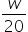Work done by B in 1 hour =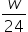Work done by C in 1 hour =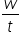Step 2 of 2:It is also given that A, B and C working together can do a piece of work in 8 hours. So we can writeWork done by A in 8 hours + Work done by B in 8 hours + Work done by C in 8 hours = w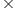8 +8 +8 = w8w(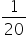+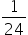+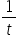) = w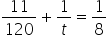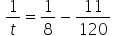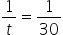t = 30 hoursFinal Answer: Hence, C alone can do the same work in 30 hours.#### With Turito Foundation.#### Get an Expert Advice From Turito.